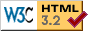## Institut für Astronomie und Astrophysik

### Abteilung Astronomie

Sand 1, D-72076 Tübingen, Germany

## Preprint 09/95

### Vertical Structure and Spectrum of Accretion Disks in Active Galactic Nuclei

T. Dörrer(1), H. Riffert(2), R. Staubert(1), and H. Ruder(2)

(1)Institut für Astronomie und Astrophysik, Astronomie, Universität Tübingen, Waldhäuser Str. 64, D-72076 Tübingen, Germany
(2)Institut für Astronomie und Astrophysik, Theoretische Astrophysik, Universität Tübingen, Auf der Morgenstelle 10, D-72076 Tübingen, Germany

accepted by Astronomy & Astrophysics

Abstract. Radiation emitted from an accretion disk around a massive black hole is a widely discussed model for the UV/soft X-ray excess emission observed in the spectra of many AGN. A self-consistent calculation of the structure and the emerging spectrum of geometrically thin alpha-accretion disks in AGN is presented. The central object is assumed to be a Kerr black hole; full relativistic corrections are included. The local dissipation of gravitational energy is assumed to be entirely due to turbulence. Since these disks are mainly supported by radiation pressure, the assumption that the viscous energy production is proportional to the total pressure leads to diverging temperature structures in the upper parts of the disk, where the total optical depths is small. We therefore modify the standard expression for the turbulent viscosity by taking into account the radiative energy loss of turbulent elements in an optically thin regime. Compton scattering is treated in the Fokker-Planck approximation using the Kompaneets oparator. The absorption cross section contains only free-free processes for a pure hydrogen atmosphere. We present several calculations for various parameters such as the accretion rate M_dot, the viscosity parameter alpha, the specific angular momentum a of the black hole and the inclination angle Theta_0 of the observer. The resulting temperature and density profiles show that the disks are optically thick for Compton scattering and effectively optically thin for most frequencies. This leads to spectra that are diluted with respect to the photon number but contain a Comptonized high energy tail in order to carry the required energy flux. In addition the electron temperature deviates strongly from the equilibrium temperature. For a model with M=10^8 M_sun, M_dot=0.3, alpha=1/3 and a/M=0.998 the fraction of total flux emitted in the soft and hard X-ray band (>2.4* 10^16 Hz) for Theta_0 = 0 , 41, 60, 70 and 90 degrees is 36%, 50%, 64%, 78% and 93%, respectively. Therefore, the model can in general account for the observed soft X--ray excess.

Key words: accretion disks - black hole physics - Galaxies: active - radiative transfer

Paper (79k gzip'ed Postscript including figures)

 Jürgen Barnstedt (barnstedt AT astro.uni-tuebingen.de) Last updated 04-Aug-1997